# RoBERTa Trained onBookCorpus, English Wikipedia, CC-News, OpenWebText and Stories Datasets

Represent text as a sequence of vectors

Released in 2019, this model uses various pre-training and design optimizations, like longer training periods on bigger batches of data and training over more data, removing next-sentence prediction objective, training on longer sequences and changing masking patterns dynamically, to obtain substantial improvement in performance over the existing BERT models.

Number of models: 3

## Performance

• Accuracy of the RoBERTa-Large model for various natural language inference tasks: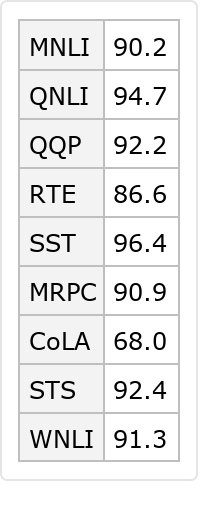## Examples

### Resource retrieval

Get the pre-trained net:

 In:=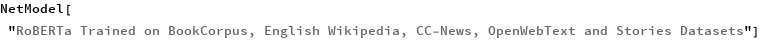Out=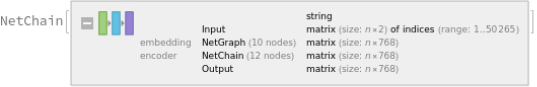### NetModel parameters

This model consists of a family of individual nets, each identified by a specific parameter combination. Inspect the available parameters:

 In:=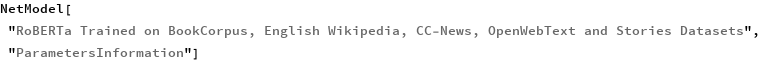Out=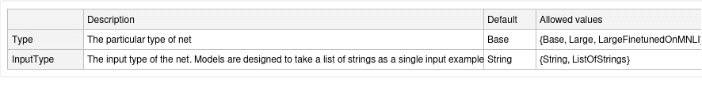Pick a non-default net by specifying the parameters:

 In:=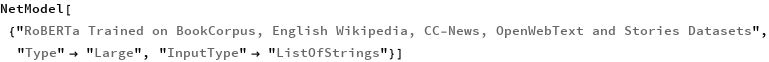Out=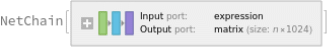Pick a non-default uninitialized net:

 In:=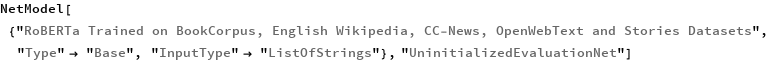Out=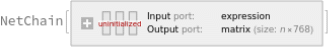### Basic usage

Given a piece of text, the RoBERTa net produces a sequence of feature vectors of size 768, which correspond to the sequence of input words or subwords:

 In:=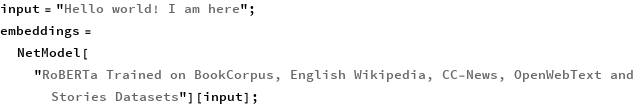Obtain dimensions of the embeddings:

 In:=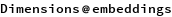Out=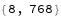Visualize the embeddings:

 In:=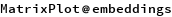Out=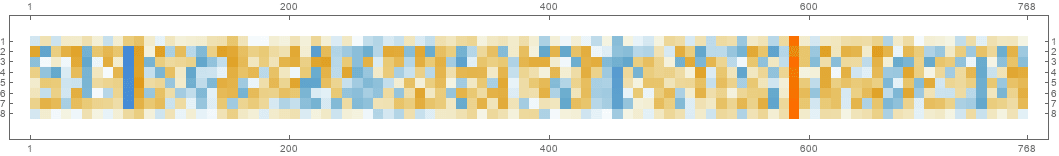### Transformer architecture

Each input text segment is first tokenized into words or subwords using a word-piece tokenizer and additional text normalization. Integer codes called token indices are generated from these tokens, together with additional segment indices:

 In:=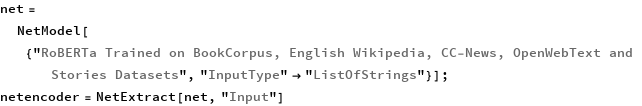Out=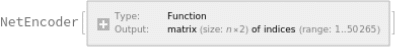For each input subword token, the encoder yields a pair of indices that correspond to the token index in the vocabulary, and the index of the sentence within the list of input sentences:

 In:=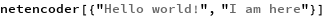Out=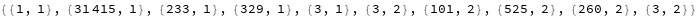The list of tokens always starts with special token index 1, which corresponds to the classification index. The special token index 3 is used as a separator between the different text segments, marking the end and beginning (except the first) of each sentence. Each subword token is also assigned a positional index:

 In:=Out=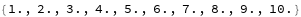A lookup is done to map these indices to numeric vectors of size 768:

 In:=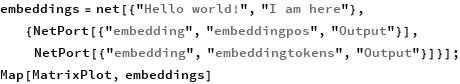Out=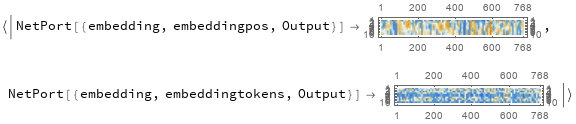For each subword token, these three embeddings are combined by summing elements with ThreadingLayer:

 In:=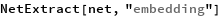Out=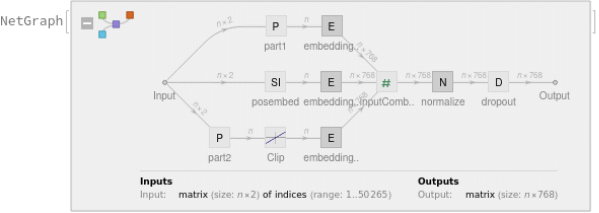The transformer architecture then processes the vectors using 12 structurally identical self-attention blocks stacked in a chain:

 In:=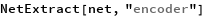Out=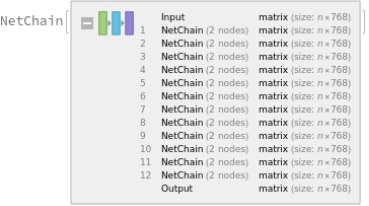The key part of these blocks is the attention module comprising of 12 parallel self-attention transformations, also called “attention heads”:

 In:=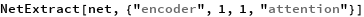Out=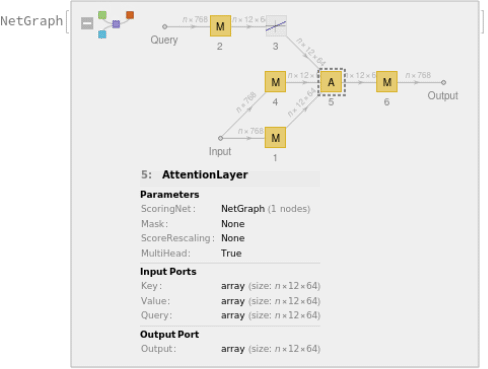BERT-like models use self-attention, where the embedding of a given subword depends on the full input text. The following figure compares self-attention (lower left) to other types of connectivity patterns that are popular in deep learning: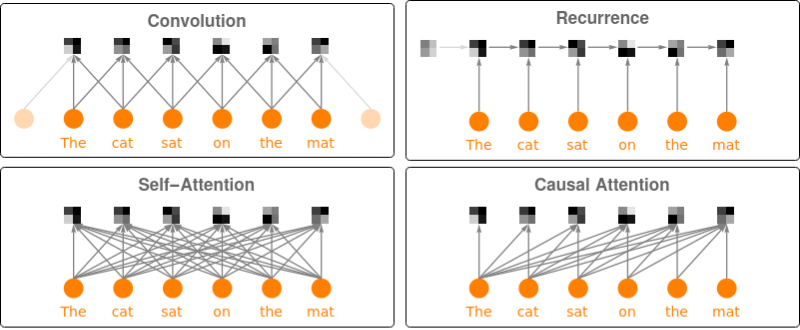### Sentence analogies

Define a sentence embedding that takes the last feature vector from RoBERTa subword embeddings (as an arbitrary choice):

 In:=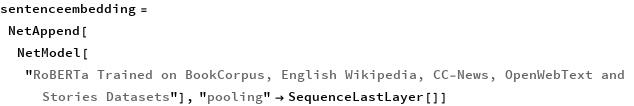Out=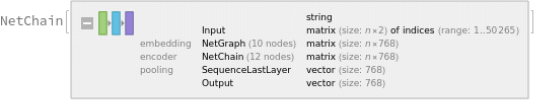Define a list of sentences in two broad categories (food and music):

 In:=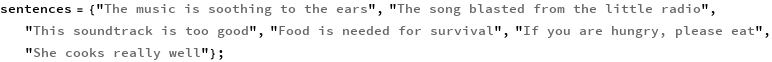Precompute the embeddings for a list of sentences:

 In:=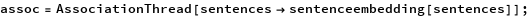Visualize the similarity between the sentences using the net as a feature extractor:

 In:=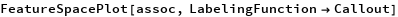Out=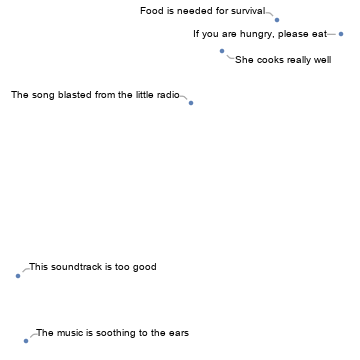### Train a classifier model with the subword embeddings

Get a text-processing dataset:

 In:=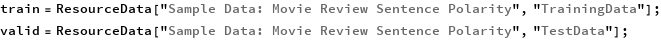View a random sample of the dataset:

 In:=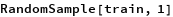Out=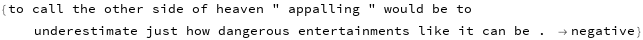Precompute the RoBERTa vectors for the training and the validation datasets (if available, GPU is highly recommended):

 In:=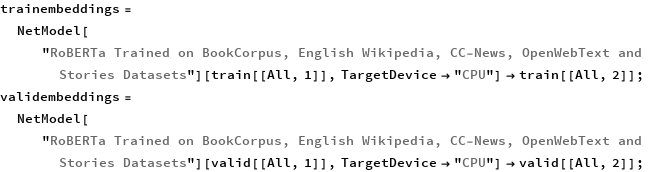Define a network to classify the sequences of subword embeddings, using a max-pooling strategy:

 In:=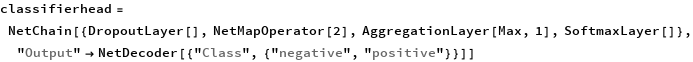Out=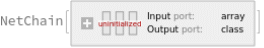Train the network on the precomputed vectors from RoBERTa:

 In:=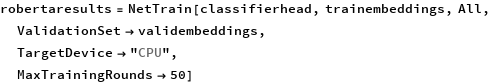Out=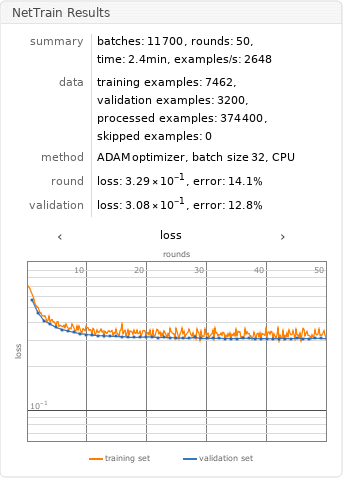Check the classification error rate on the validation data:

 In:=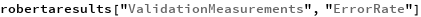Out=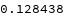Let’s compare the results with the performance of a classifier trained on context-independent word embeddings. Precompute the GloVe vectors for the training and the validation dataset:

 In:=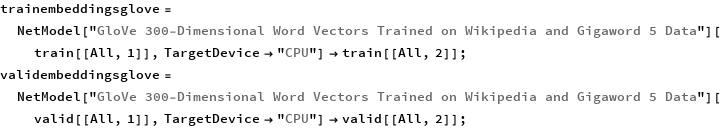Train the classifier on the precomputed GloVe vectors:

 In:=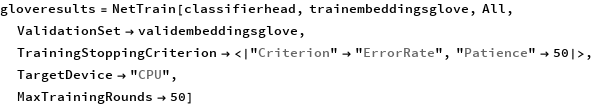Out=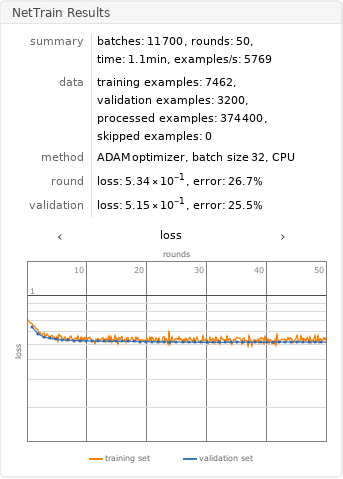Compare the results obtained with RoBERTa and with GloVe:

 In:=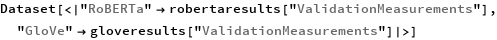Out=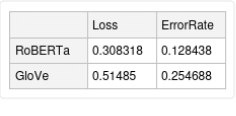### Net information

Inspect the number of parameters of all arrays in the net:

 In:=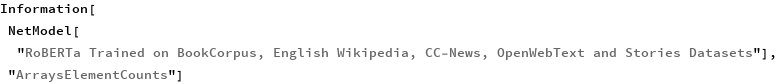Out=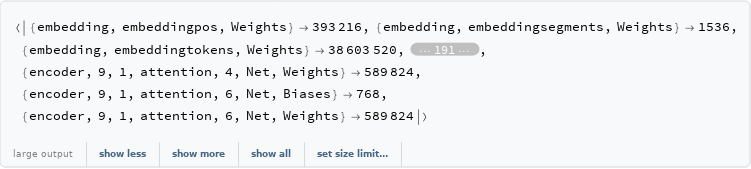Obtain the total number of parameters:

 In:=Out=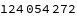Obtain the layer type counts:

 In:=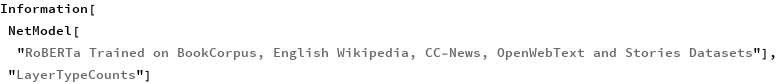Out=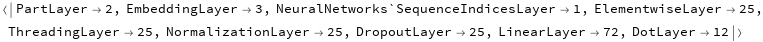Display the summary graphic:

 In:=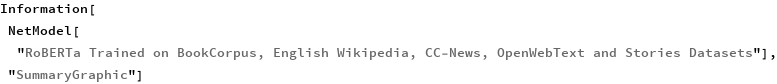Out=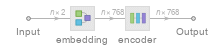### Export to MXNet

Export the net into a format that can be opened in MXNet:

 In:=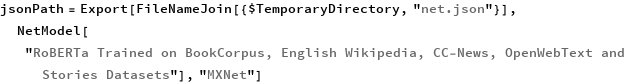Out=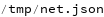Export also creates a net.params file containing parameters:

 In:=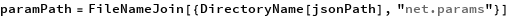Out=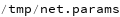Get the size of the parameter file:

 In:=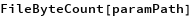Out=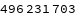## Requirements

Wolfram Language 12.1 (March 2020) or above